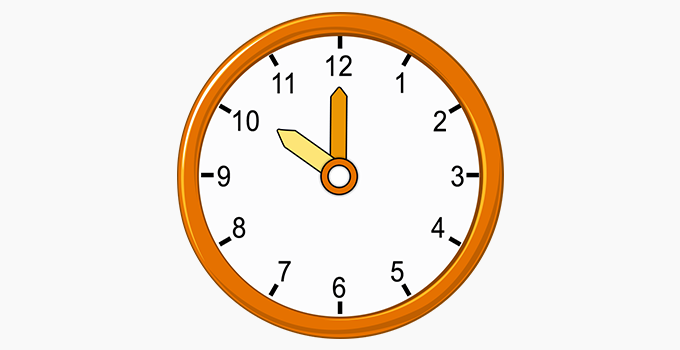# Subtracting Time - Definition with Examples

The Complete K-5 Math Learning Program Built for Your Child

• 30 Million Kids

Loved by kids and parent worldwide

• 50,000 Schools

Trusted by teachers across schools

• Comprehensive Curriculum

Aligned to Common Core

## Subtracting Time

Time tells us about the duration of a task or an event.

We measure time in hours, minutes, and seconds. There are 60 minutes in an hour and 60 seconds in a minute.## Subtracting hours and minutes

Here are the steps to subtract time (4 hours 45 minutes - 2 hours 20 minutes) given in hours and minutes.

Step I: Check if the minutes of the first time (4 hours 45 minutes) is less than the minutes of the second time (2hour 20 minutes). If that is the case then,

• Take one away from the hours of the first time.
• Add 60 to the minutes of the first time.

(Since 45 is not less than 20, we move on to the next step)

Step II: Subtract the minutes. (45 minutes - 20 minutes = 25 minutes)

Step III: Subtract the hours. (4 hours - 2 hours = 2 hours)

Hence, the resultant from Step II and Step III together is the answer (2hours 25minutes).

Example 1: Solve the following

• 7 hours 45 minutes – 6 hours and 15 minutes.
• 7 hours 15 minutes – 6 hours and 45 minutes

Solution:

A.

Step I: Since 45 is not less than 15, we move on to the next step

Step II: On taking away the minutes, we get,

45 minutes
– 15 minutes
30 minutes

Step III: On taking away the hours, we get,

7 hours
– 6 hours
1 hour

Hence, the answer is 1 hour and 30 minutes.

B.

Step I: Since 15 is less than 45, we take one away from the hours of the first time and add 60 to the minutes of the first time.

• 7 – 1 = 6 hour
• 15 + 60 = 75minutes

Step II: On taking away the minutes, we get,

75 minutes
– 45 minutes
30 minutes

Step III: On taking away the hours, we get,

6 hours
– 6 hours
0 hours

Hence, the answer is 0 hours 30 minutes or just 30 minutes.

## Clock

A clock is a device we use to measure time. Time on a clock tells us when a certain event occurs or finishes.

For example, the clock shows a time of 7 a.m.Given below is a clock, the bigger hand is the minute hand and the shorter hand is the hour hand. The red-colored hand is the second hand.## Subtracting Clock Time

Here are the steps to subtract two times of a clock (4:45 pm – 2:20 pm):

Case I: If both the times are either in am or in pm

Step I: Check if the minutes of the first time (2:45 pm) is less than the minutes of the second time (2:20 pm). If that is the case then

• Take one away from the hours of the first time.
• Add 60 to the minutes of the first time.

(Since 45 is not less than 20, we move on to the next step)

Step II: Subtract the minutes. (45 - 20 = 25 minutes)

Step III: Subtract the hours. (4 - 2 = 2 hours)

Hence, the resultant from Step II and Step III together is the answer (2 hours 25 minutes).

Example 2: Solve the following

A: 8:15 pm-6:45pm

Solution:

A. 8:15 pm-6:45pm

Step I: Since 15 is less than 45, we take one away from the hours of the first time and add 60 to the minutes of the first time.

8 - 1 = 7 hour

15 + 60 = 75 minutes

Step II: On taking away the minutes, we get,

75 minutes
– 45 minutes
30 minutes

Step III: On taking away the hours, we get,

7  hours
- 6  hours
1 hour

Hence, the answer is 1 hour and 30 minutes.

Case II: If one of the times is in am and the other is in pm (8:30 am – 2:10 pm), we will follow the below steps:

Step I: Take away hours of the first time (8:30 am) from 11 (11 – 8 = 3 hours) and also take away minutes of the first time from 60 (60 - 30 = 30 minutes).

Step II: Add hours obtained from Step I (3 hours) and hours of the second time (2:10 pm).

(3+2 = 5 hours)

Step III: Add minutes obtained from Step I (30 minutes) and minutes of the second time (2:10 pm).

(30 + 10 = 40minutes)

The answer to Step II and III together is our final answer (5 hours 40 minutes).

Example 3: Solve 10: 45 am – 2: 15 pm.

Solution:

Since one of the time is in am and the other time is in pm, steps of case II will be used.

Step I: On taking away hour and minutes of 10:45 am from 11 and 60 respectively, we get,

11 – 10 = 1 hour

60 – 45 = 15 minutes

Step II: On adding hours of 2:15 pm and hours obtained from step I, we get

1 + 2 = 3 hours

Step III: On adding minutes of 2:15 pm and minutes obtained from step I, we get,

15 + 15 = 30 min

Hence, the answer is 3 hours and 30 minutes.

## Fun Fact-

• Every day we go from our past to the future through the present, which makes us time travelers.

Won Numerous Awards & Honors